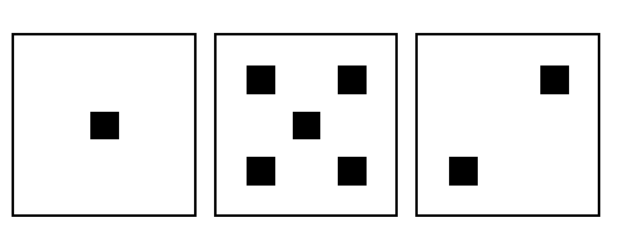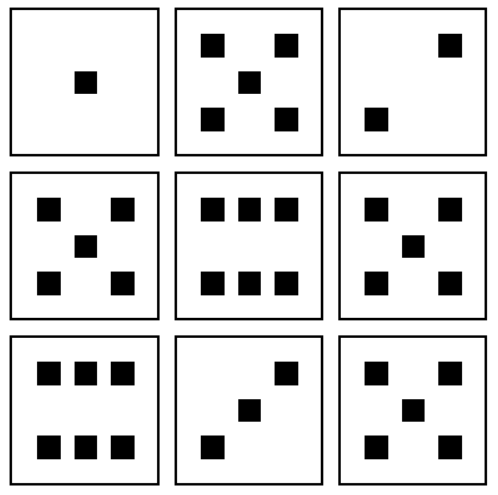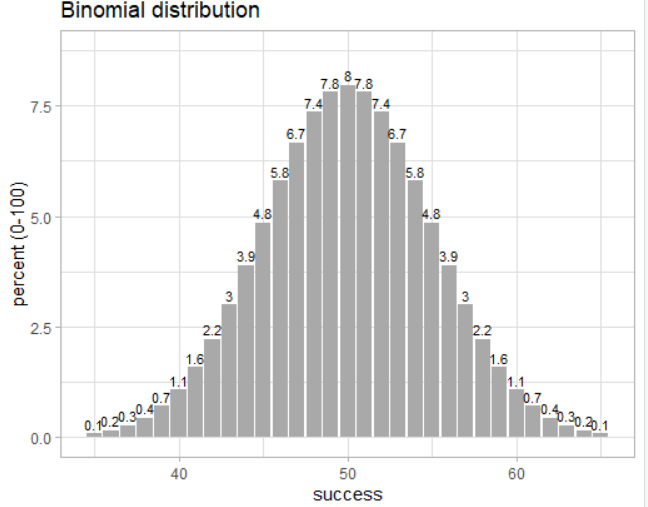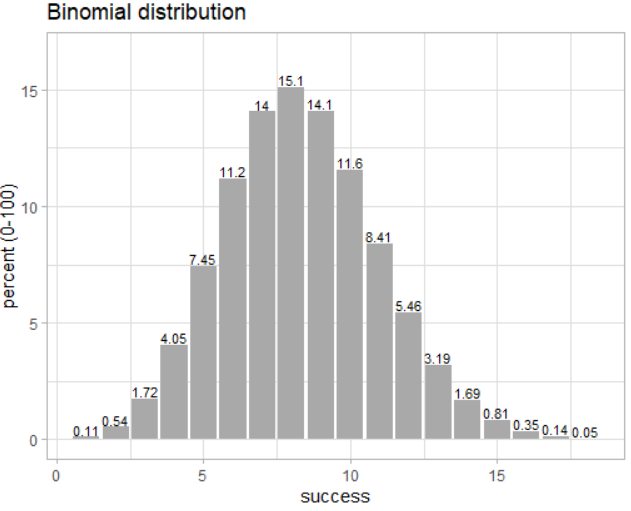Chhaya Wagmi

# Explore R Libraries: tidydice

• Sep 14, 2020
• 242 Views
• Sep 14, 2020
• 242 Views
Data
Data Analytics
Machine Learning
R

## Introduction

When you were in high school, you probably studied basic statistics using virtual experiments like flipping a coin, rolling dice, or selecting a certain number of cards. When you start your career in data analysis, you will frequently encounter various situations where you might need to recall those experiments to help you learn various statistical topics, such as probability distributions, moments, the law of large numbers, and so on. If you are an R user, you can benefit from the tidydice library, which presents automated results of rolling a die and flipping a coin.

In this guide, you will learn to perform these simulations and plot their binomial distribution.

## Basics

``1install.packages("tidydice")``
r

`package ‘tidydice’ successfully unpacked and MD5 sums checked`

Next, load the package in your current R environment using the given code:

``1library(tidydice)``
r

Note: You can also install and load the tidyverse package, which goes hand in hand with the tidydice package.

## Simulating Experiments Related to Flipping of a Coin

The tidydice package provides the `flip_coin` function with which you can conduct experiments. The code below, from R’s documentation, demonstrates a few of the possible variations of the `flip_coin` function.

``````1flip_coin(data = NULL, times = 1, rounds = 1, success = c(2),
2  agg = FALSE, sides = 2, prob = NULL, seed = NULL)
``````

The results are not expressed as Heads and Tails, but rather 1 and 2. By default, the coin is unbiased, but you can change its behavior by using the `prob` parameter. The code below demonstrates a few of the possible variations of the `flip_coin` function.

``````1# 1. Flipping a coin two times
2flip_coin(times = 2)
3
4# # A tibble: 2 x 5
5# experiment round    nr result success
6# <int> <int> <int> <int> <lgl>
7#   1     1     1      1 FALSE
8#   1     1     2      2 TRUE
9
10
11# 2. Flipping a coin two times for three rounds
12flip_coin(times = 2, rounds = 3)
13
14# # A tibble: 6 x 5
15# experiment round    nr result success
16# <int> <int> <int> <int> <lgl>
17#  1     1     1      2 TRUE
18#  1     1     2      1 FALSE
19#  1     2     1      1 FALSE
20#  1     2     2      1 FALSE
21#  1     3     1      2 TRUE
22#  1     3     2      2 TRUE
23
24
25# 3. Flipping a coin three times by inducing bias
26#     of 90% to one of the side
27s
28flip_coin(times = 3, prob = c(0.1, 0.9))
29
30# # A tibble: 3 x 5
31# experiment round    nr result success
32# <int> <int> <int> <int> <lgl>
33# 1     1     1      1 FALSE
34# 1     1     2      2 TRUE
35# 1     1     3      2 TRUE
36
37
38# 4. Flipping a coin 5 times and reproducing
39#     the same results with every run
40flip_coin(times = 5, seed = 42)
41
42# # A tibble: 5 x 5
43# experiment round    nr result success
44# <int> <int> <int> <int> <lgl>
45# 1     1     1      1 FALSE
46# 1     1     2      1 FALSE
47# 1     1     3      1 FALSE
48# 1     1     4      1 FALSE
49# 1     1     5      2 TRUE
50
51
52# 5. Combining experiments of flipping coins
53flip_coin(times = 3, seed = 41, agg = T) %>%
54  flip_coin(times = 2, seed = 42, agg = T)
55
56# # A tibble: 2 x 4
57# experiment round times success
58# <int> <int> <int> <int>
59# 1     1     3       1
60# 2     1     2       0``````
r

## Simulating Experiments Related to Rolling a Die

The tidydice package provides great flexibility in simulating experiments of rolling a die by giving you not only a textual representation of results, but also graphical ones. The code below, from R’s documentation, demonstrates a few of the possible variations of the `roll_dice` function.

``````1roll_dice(data = NULL, times = 1, rounds = 1, success = c(6),
2  agg = FALSE, sides = 6, prob = NULL, seed = NULL)
``````

The parameters of this function are similar to that of the `flip_coin` function. By default, the die is fair and you can bring bias to any of the sides using the `prob` parameter. Also by default, `success` is set to side 6, so you will receive `TRUE` when the dice results in the value 6. You can update this information anytime.

Here are two experiments based on this function:

``````1# 1. Rolling a die four times with 50% bias to its third side
2#    and 10% to the rest of the sides. Also, providing a seed value
3#    for reproducible results
4
5roll_dice(times = 4, prob = c(0.1, 0.1, 0.5, 0.1, 0.1, 0.1), seed = 100)
6
7# # A tibble: 4 x 5
8# experiment round    nr result success
9# <int> <int> <int>  <int> <lgl>
10#   1     1     1      3   FALSE
11#   1     1     2      3   FALSE
12#   1     1     3      6   TRUE
13#   1     1     4      3   FALSE
14
15# 2. Rolling a die 10 times and finding how many times
16#    the second side is rolled by setting the parameter 'success' to 2
17
18roll_dice(times = 10, agg = TRUE, success = c(2), seed = 100)
19
20# # A tibble: 1 x 4
21# experiment round times success
22# <int> <int> <int>   <int>
23#   1     1    10       2``````
r

So far, you have learned how to generate tabular results of rolling a die. You can also get these results as a plot to make simulations more pleasant to the eye:

``````1# 1. Rolling a die 3 times with a seed value
2roll_dice(times = 3, seed = 402) %>%
3  plot_dice()``````
r``````1# 2. Rolling a die 3 times in 3 rounds with a seed value
2roll_dice(times = 3, rounds = 3, seed = 402) %>%
3  plot_dice()``````
r## Plotting a Binomial Distribution of your Experiment

You can plot the binomial distribution of your experiment using the command `plot_binom`.

Binomial distribution of flipping a coin 100 times:

``1binom_coin(times = 100) %>% plot_binom()``
rBinomial distribution of rolling a die 50 times:

``1binom_dice(times = 50) %>% plot_binom()``
r## Conclusion

In this guide, you learned how to create automated simulations related to flipping a coin and rolling a die (including creating a plot). You can use these simulations with other statistical packages in R or to revise your statistical concepts.